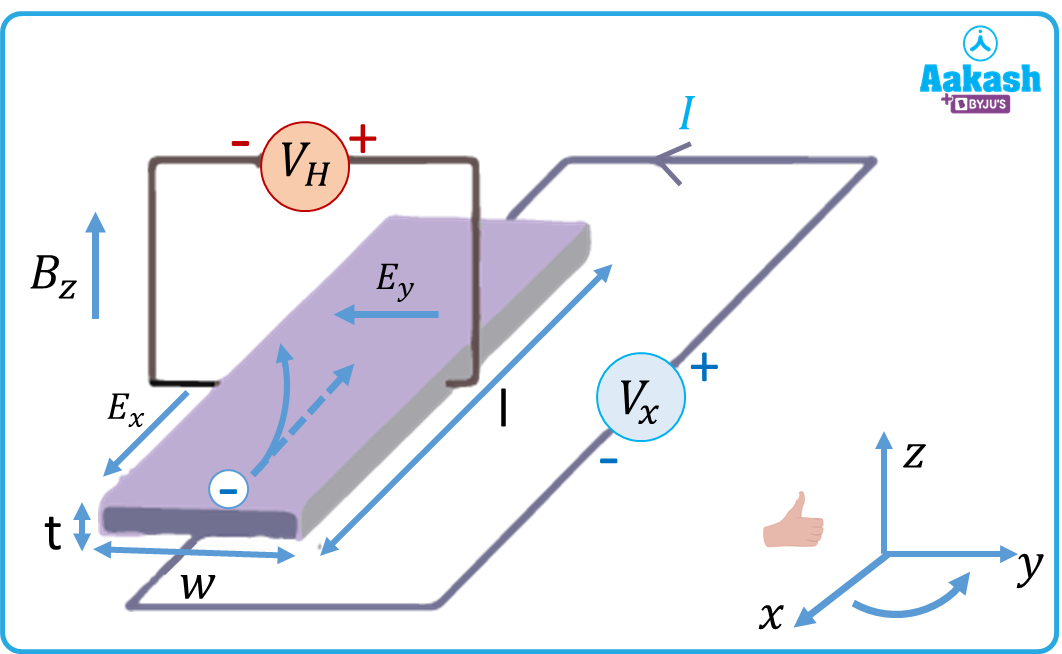• Call Now

1800-102-2727•

# Hall effect - Definition, Illustrations, Practice problems, FAQs

The Hall effect is observed in a conductor whenever it is falling in a region where both magnetic and electric fields are present. Scientist Hendrick Lorentz proposed the concept of Lorentz force, which states that the charges in a conductor experience forces due to both electric and magnetic fields. Edwin Hall, in 1879, observed that a potential difference is developed between the ends of a current carrying conductor that is falling in a magnetic field. It is also applicable for semiconductors. In this article, we will explore the Hall effect in detail.

• Definition of Hall effect
• Theory of Hall effect
• Hall coefficient
• Applications of Hall effect
• Practice problems
• FAQs

## Definition of Hall effect

When a solid material, carrying an electric current, is placed in a magnetic field, then an electric field is set up in a transverse direction inside the material. The principle of Hall effect is that when a semiconductor or a conductor is placed perpendicular to the magnetic field, then an appreciable voltage or potential difference is established between the ends of the material, which is in turn called the Hall effect. When no magnetic field is applied, the charge carriers travel in a straight path without being deflected. This straight line is called the “line of sight path”. On the other hand, when a magnetic field is applied, the path of the charge carries becomes curved. Due to this curved trajectory, the charges accumulate on either side of the conductor - the positive charges towards one side, and the negative charges towards the other. Hence, the charge gets distributed symmetrically across the conductor.

## Theory of Hall effect

When a conductive plate is placed between the ends of a battery, then the circuit gets completed, and the current starts flowing. The charge flows in a linear path from one end of the plate to the other end. This motion of charge carriers causes the production of a magnetic field. When a magnet is brought near the plate, it distorts the existing magnetic field. The force exerted on the charge carries is nothing but the Lorentz force.

As a result of the distortion, the negatively charged electrons get thrown towards one side of the plate, and the positive holes towards the other. The Hall voltage thus established can be written as $,{V}_{H}=\frac{{I}_{x}{B}_{z}}{nte}$

n- number density of charge carriers per unit volume
Bz- magnetic field strength
e- value of electronic charge

Also,

VH= VxBzw
Vx-Voltage across the conductor
Bz- Magnetic field
w- width of the conductor
tw- cross sectional areaNote:

The charge carriers could be ions, electrons, holes or maybe even a combination of the three.

## Hall coefficient

The Hall coefficient RH can be written as,

${R}_{H}=\frac{{E}_{y}}{{J}_{x}{B}_{z}}$

Jx- current density
Ey- Electric field established due to Hall Effect
Bz- Magnetic field

The Hall coefficient is positive if the number of positive charges is greater than the number of negative charges.

, being the conductivity. e is mobility.

## Applications of Hall effect

Hall effect finds applications in the following areas:

1) Proximity sensors
2) Hall sensors and Hall probes
3) Linear and angular displacement transducers
4) Phase angle measurement

## Practice problems

Q. Calculate the Hall voltage when a conductor carrying a current of 100 A, is placed in a magnetic field of 1.5 T. The conductor has a thickness of 1 cm, and the number density of charges inside the conductor is

A.

Q. The width of a conductor is 2.0 10-2 m. The voltage across its ends is 5 V. It is made to fall freely inside a magnetic field whose magnitude is 0.2 T. Calculate the Hall voltage between the ends of the conductor.

A.

Given,

Q. The current density inside a conductor is 2 104 Am-2. The electric field in the region is 104 N/C and the magnetic field is 0.1 T. Calculate the Hall coefficient.

A.

Given,

${R}_{H}=$

Q. An electric field of 100 V/m is applied to an n- type semiconductor whose Hall coefficient is 0.0125 m3/C. Calculate the current density, if mobility is 0.6 m2 V/s.

A.

Given,

e= RH, being the conductivity.

## FAQs

Q. Can the Hall effect be observed in conductors?
A.
In most conductors, the Hall effect is observed only to a small extent, since the density of electrons is very high.

Q. Which parameter cannot be determined from Hall’s effect?
A.
The hall effect cannot be used to determine the magnetic field, which does not act perpendicular to the direction of the electric field.

Q. Why is the Hall effect more pronounced in semiconductors?
A.
The density of charge carriers is less in semiconductors. Since Hall voltage is inversely proportional to the number of charge carriers, it is more effective in the case of semiconductors.

Q. Can the Hall coefficient be zero?
A.
The Hall coefficient depends upon the hole/electron concentration, and also on the mobility of carriers. In an insulator, the gap between conduction and valence band is very high. In insulators, the conductivity is zero, hence, mobility is close to zero as well. Hence the Hall effect is zero in the case of an insulator.Talk to our expert
Resend OTP Timer =
By submitting up, I agree to receive all the Whatsapp communication on my registered number and Aakash terms and conditions and privacy policy